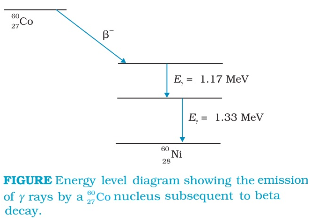### Gamma Radiations:

There are energy levels in a nucleus, just like there are energy levels in atoms. When a nucleus is in an excited state, it can make a transition to a lower energy state by the emission of electromagnetic radiation. As the energy differences between levels in a nucleus are of the order of MeV, the photons emitted by the nuclei have MeV energies and are called gamma rays.### Units of Radioactive Decay

1 Becquerel = 1Bq = 1 decay per second

1 Rutherford = 1 rd = 106 decay per second

1 curie = 1 Ci = 3.7 × 1010 decays per second

### Mass-Energy Equivalence

Einstein showed from his theory of special relativity that it is necessary to treat mass as another form of energy.

Einstein gave the famous mass-energy equivalence relation

E = mc2

Here the energy equivalent of mass m is related by the above equation and c is the velocity of light in vacuum and is approximately equal to 3×108 ms-1.